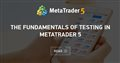# Why would this work perfectly in strategy tester, but absolutely makes no sense in live?

So this code opens up a trade based on risk I set on EA options. Say I set the acc size to 1000 and risk to 1. That means the EA will risk 100\$. SL should be at -100\$. and TP is 1:1 so at +100\$- lot size.will be calculated automatically. It works perfectly in Strategy tester. But on live the SL would be at like -2\$ and completely wrong Lot size and TP is far away but still +100\$. So anyone knows why is this happening.
```void openBuy(double openPrice, double stoploss, double takeprofit)
{
}

void openSell(double openPrice, double stoploss, double takeprofit)
{
}

void openTrade(int type, double openPrice, double stoploss, double takeprofit)
{
double tickSize = MarketInfo(Symbol(), MODE_TICKSIZE);
double tickCount = MathAbs(openPrice-stoploss)/tickSize;
double tickValue = MarketInfo(Symbol(), MODE_TICKVALUE);
double valueToRisk = acc_size * risk_perc / 100;
double positionSize = (tickCount * tickValue) != 0 ? valueToRisk/(tickCount * tickValue) : 0;
Print(positionSize);
positionSize = NormalizeDouble(positionSize, 2);
if(positionSize == 0)
{
Print("error while calculating lot size");
Print(openPrice);
Print(stoploss);
Print(tickCount);
Print(tickValue);
Print(tickSize);
Print(valueToRisk);
Print(tickCount * tickValue);
Print(MathAbs(openPrice - stoploss)/_Point/10);
}
else
{
if(type == 1)
{
if(!OrderSend(Symbol(), OP_BUY, positionSize, openPrice,50, stoploss, takeprofit))
{
Print(GetLastError());
}
}
else
{
if(!OrderSend(Symbol(), OP_SELL, positionSize, openPrice, 50, stoploss, takeprofit))
{
Print(GetLastError());
}
}
}
}```The Fundamentals of Testing in MetaTrader 5
• www.mql5.com
What are the differences between the three modes of testing in MetaTrader 5, and what should be particularly looked for? How does the testing of an EA, trading simultaneously on multiple instruments, take place? When and how are the indicator values calculated during testing, and how are the events handled? How to synchronize the bars from different instruments during testing in an "open prices only" mode? This article aims to provide answers to these and many other questions.
Reason: